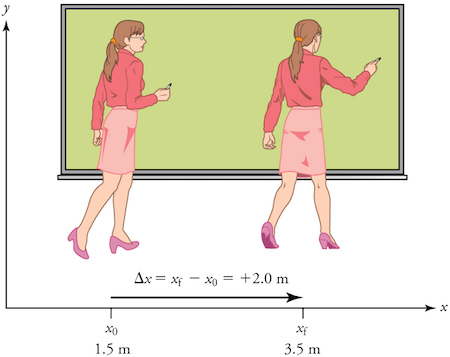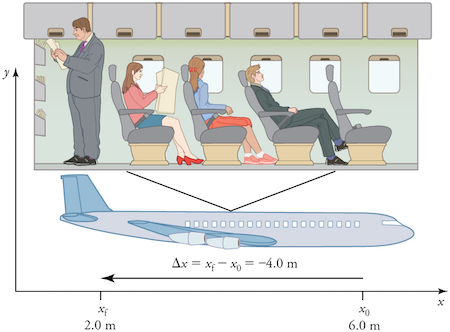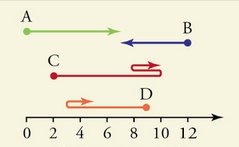• # What is displacement?

Organizer
April 12, 2021 at 10:51 pm

What does position mean?

In physics, we love to precisely describe the motion of an object. Seriously, the first few chapters of basically every physics textbook are devoted to teaching people how to precisely describe motion since it is so important to everything else we do in physics.

But to describe an object’s motion, we have to first be able to describe its position—where it is at any particular time. More precisely, we need to specify its position relative to a convenient reference frame. Earth is often used as a reference frame, and we often describe the position of an object as it relates to stationary objects in that reference frame. For example, a professor’s position could be described in terms of where she is in relation to the nearby whiteboard (Figure 1). In other cases, we use reference frames that are not stationary but rather are in motion relative to Earth. To describe the position of a person in an aeroplane, for example, we use the aeroplane, not Earth, as the reference frame (Figure 2).

The variable xxx is often used to represent the horizontal position. The variable yyy is often used to represent the vertical position.

What does displacement mean?

If an object moves relative to a reference frame—for example, if a professor moves to the right relative to a whiteboard, or a passenger moves toward the rear of an aeroplane—then the object’s position changes. This change in position is known as displacement. The word displacement implies that an object has moved, or has been displaced.

Displacement is defined to be the change in the position of an object. It can be defined mathematically with the following equation:

\text{Displacement}=\Delta x=x_f-x_0Displacement=Δx=xf​−x0​start text, D, i, s, p, l, a, c, e, m, e, n, t, end text, equals, delta, x, equals, x, start subscript, f, end subscript, minus, x, start subscript, 0, end subscript

x_fxf​x, start subscript, f, end subscript refers to the value of the final position.
x_0x0​x, start subscript, 0, end subscript refers to the value of the initial position.
\Delta xΔxdelta, x is the symbol used to represent displacement.

[What does the triangle symbol mean?]

Displacement is a vector. This means it has a direction as well as a magnitude and is represented visually as an arrow that points from the initial position to the final position. For example, consider the professor that walks relative to the whiteboard in Figure 1.Figure 1: A professor paces left and right while lecturing. The +2.0\text{ m}+2.0 mplus, 2, point, 0, start text, space, m, end text displacement of the professor relative to the whiteboard is represented by an arrow pointing to the right. (Image credit: Openstax College Physics)

The professor’s initial position is x_0=1.5\text{ m}x0​=1.5 mx, start subscript, 0, end subscript, equals, 1, point, 5, start text, space, m, end text and her final position is x_f=3.5\text{ m}xf​=3.5 mx, start subscript, f, end subscript, equals, 3, point, 5, start text, space, m, end text. Thus, her displacement can be found as follows, \Delta x=x_f−x_0=3.5 \text{ m}−1.5\text{ m}=+2.0 \text{ m}Δx=xf​−x0​=3.5 m−1.5 m=+2.0 mdelta, x, equals, x, start subscript, f, end subscript, −, x, start subscript, 0, end subscript, equals, 3, point, 5, start text, space, m, end text, −, 1, point, 5, start text, space, m, end text, equals, plus, 2, point, 0, start text, space, m, end text. In this coordinate system, motion to the right is positive, whereas motion to the left is negative.

Now consider the passenger that walks relative to the plane in Figure 2.Figure 2: A passenger moves from his seat to the back of the plane. The −4.0\text{ m}−4.0 m−, 4, point, 0, start text, space, m, end text displacement of the passenger relative to the plane is represented by an arrow toward the rear of the plane. (Image credit: Openstax College Physics)

The airplane passenger’s initial position is x_0=6.0\text{ m}x0​=6.0 mx, start subscript, 0, end subscript, equals, 6, point, 0, start text, space, m, end text and his final position is x_f=2.0\text{ m}xf​=2.0 mx, start subscript, f, end subscript, equals, 2, point, 0, start text, space, m, end text, so his displacement can be found as follows, \Delta x=x_f−x_0=2.0 \text{ m}−6.0\text{ m}=-4.0 \text{ m}Δx=xf​−x0​=2.0 m−6.0 m=−4.0 mdelta, x, equals, x, start subscript, f, end subscript, −, x, start subscript, 0, end subscript, equals, 2, point, 0, start text, space, m, end text, −, 6, point, 0, start text, space, m, end text, equals, minus, 4, point, 0, start text, space, m, end text. His displacement is negative because his motion is toward the rear of the plane, or in the negative x direction in our coordinate system.

In one-dimensional motion, direction can be specified with a plus or minus sign. When you begin a problem, you should select which direction is positive—usually that will be to the right or up, but you are free to select positive as being any direction.

What do distance and distance traveled mean?

We must be careful when using the word distance since there are two ways in which the term distance is used in physics. We can talk about the distance between two points, or we can talk about the distance traveled by an object.

Distance is defined to be the magnitude or size of displacement between two positions. Note that the distance between two positions is not the same as the distance traveled between them.

Distance traveled is the total length of the path traveled between two positions. Distance traveled is not a vector. It has no direction and, thus, no negative sign. For example, the distance the professor walks is 2.0 \text{ m}2.0 m2, point, 0, start text, space, m, end text. The distance the airplane passenger walks is 4.0 \text{ m}4.0 m4, point, 0, start text, space, m, end text.

It is important to note that the distance traveled does not have to equal the magnitude of the displacement (i.e., distance between the two points). Specifically, if an object changes direction in its journey, the total distance traveled will be greater than the magnitude of the displacement between those two points. See the solved examples below.

People often forget that the distance traveled can be greater than the magnitude of the displacement. By magnitude, we mean the size of the displacement without regard to its direction (i.e., just a number with a unit). For example, the professor could pace back and forth many times, perhaps walking a distance of 150 meters during a lecture, yet still end up only two meters to the right of her starting point. In this case, her displacement would be +2 +2 plus, 2, start a text, space, m, end text, the magnitude of her displacement would be 2 \ texts { m}2 m2, start a text, space, m, end text, but the distance she travelled would be 150 \ m}150 m150, start a text, space, m, end text. In kinematics, we nearly always deal with displacement and magnitude of displacement and almost never with distance travelled. One way to think about this is to assume you marked the start of the motion and the end of the motion. The displacement is simply the difference in the position of the two marks and is independent of the path taken when travelling between the two marks. The distance traveled, however, is the total length of the path taken between the two marks.

People often forget to include a negative sign, if needed, in their answer for displacement. This sometimes occurs if they accidentally subtract the final position from the initial position rather than subtracting the initial position from the final position.

What do solved examples involving displacement look like?

Example 1: Displacement of four moving objects

Four objects move according to the paths shown in the diagram below. Assume the units of the horizontal scale are given in meters. (Image credit: altered from Openstax College Physics)

What was the displacement of each object?Object A had an initial position of 0\text{ m}0 m0, start text, space, m, end text and a final position of 7\text{ m}7 m7, start text, space, m, end text. The displacement of object A can be shown with this equation:

\Delta x_A= 7\text{ m}-0\text{ m}=+7\text{ m}ΔxA​=7 m−0 m=+7 mdelta, x, start subscript, A, end subscript, equals, 7, start text, space, m, end text, minus, 0, start text, space, m, end text, equals, plus, 7, start text, space, m, end text

Object B had an initial position of 12\text{ m}12 m12, start text, space, m, end text and a final position of 7\text{ m}7 m7, start text, space, m, end text. The displacement of object B can be shown with this equation:

\Delta x_B= 7\text{ m}-12\text{ m}=-5\text{ m}ΔxB​=7 m−12 m=−5 mdelta, x, start subscript, B, end subscript, equals, 7, start text, space, m, end text, minus, 12, start text, space, m, end text, equals, minus, 5, start text, space, m, end text

Object C had an initial position of 2\text{ m}2 m2, start text, space, m, end text and a final position of 10\text{ m}10 m10, start text, space, m, end text. The displacement of object C can be shown with this equation:

\Delta x_C= 10\text{ m}-2\text{ m}=+8\text{ m}ΔxC​=10 m−2 m=+8 mdelta, x, start subscript, C, end subscript, equals, 10, start text, space, m, end text, minus, 2, start text, space, m, end text, equals, plus, 8, start text, space, m, end text

Object D had an initial position of 9\text{ m}9 m9, start text, space, m, end text and a final position of 5\text{ m}5 m5, start text, space, m, end text. The displacement of object D can be shown with this equation:

\Delta x_D= 5\text{ m}-9\text{ m}=-4\text{ m}ΔxD​=5 m−9 m=−4 mdelta, x, start subscript, D, end subscript, equals, 5, start text, space, m, end text, minus, 9, start text, space, m, end text, equals, minus, 4, start text, space, m, end text

Example 2: Distance traveled of four moving objects

Four objects move according to the paths shown in the diagram below. Assume the units of the horizontal scale are given in meters. (Image credit: altered from Openstax College Physics)

What was the total distance traveled by each object?Object A travels a total distance of 7\text{ m}7 m7, start text, space, m, end text.

Object B travels a total distance of 5\text{ m}5 m5, start text, space, m, end text.

Object C travels a total distance of 8\text{ m}+2\text{ m}+2\text{ m}=12\text{ m}8 m+2 m+2 m=12 m8, start text, space, m, end text, plus, 2, start text, space, m, end text, plus, 2, start text, space, m, end text, equals, 12, start text, space, m, end text.

Object D travels a total distance of 6\text{ m}+2\text{ m}=8\text{ m}6 m+2 m=8 m6, start text, space, m, end text, plus, 2, start text, space, m, end text, equals, 8, start text, space, m, end text.